##### International Journal of
###### eISSN: 2576-4454# HydrologyMini Review Volume 2 Issue 5

# Experiment on application of hydro-plane type removable breakwater as tsunami energy dissipater for river gate

#### Tetsuya Hiraishi,1 function clickButton(){ var name=document.getElementById('name').value; var descr=document.getElementById('descr').value; var unCopyslNo=document.getElementById('unCopyslNo').value; document.getElementById("mydiv").style.display = "none"; $.ajax({ type:"post", url:"https://medcraveonline.com/captchaCode/server_action", data: { 'name' :name, 'descr' :descr, 'unCopyslNo': unCopyslNo }, cache:false, success: function (html) { //alert('Data Send');$('#msg').html(html); } }); return false; } Verify Captcha Regret for the inconvenience: we are taking measures to prevent fraudulent form submissions by extractors and page crawlers. Please type the correct Captcha word to see email ID.function refreshPage(){ $("#mydiv").load(location.href + " #mydiv"); }$(document).ready(function () { //Disable cut copy paste $('#msg').bind('cut copy paste', function (e) { e.preventDefault(); }); //Disable mouse right click$("#msg").on("contextmenu",function(e){ return false; }); }); .noselect { -webkit-touch-callout: none; /* iOS Safari */ -webkit-user-select: none; /* Safari */ -khtml-user-select: none; /* Konqueror HTML */ -moz-user-select: none; /* Firefox */ -ms-user-select: none; /* Internet Explorer/Edge */ user-select: none; /* Non-prefixed version, currently supported by Chrome and Opera */ cursor: none; } Ryoukei Azuma,2 Hideaki Handa,3 Tadao ITO4

1Disaster Prevention Research Institute, Kyoto University, Kyoto, Japan
2Osaka Institute of Technology, Osaka, Japan
3Marushima Aqua System Co. Ltd. Nara, Japan
4Marushima Aqua System Co. Ltd., Osaka, Japan

Correspondence: Tetsuya Hiraishi, Disaster Prevention Research Institute, Kyoto University, Kyoto, Japan

Received: September 27, 2018 | Published: October 3, 2018

Citation: Hiraishi T, Azuma R, Handa H, et al. Experiment on application of hydro-plane type removable breakwater as tsunami energy dissipater for river gate. Int J Hydro. 2018;2(5):573-577. DOI: 10.15406/ijh.2018.02.00128

# Abstract

The paper proposes application of the hydro-plane type removable breakwater as the tsunami energy dissipater. A series of model experiments with a scale of 1/33 are conducted employing a visor-gate type river one and hydro-plane type breakwater changing the height of drift from 0 to 1.2 times higher than the initial water level. The effect of tsunami energy dissipating becomes the highest when the breakwater is adjusted to drift up until the initial water surface level. Meanwhile the dissipating effect increased as the initial tsunami height increased. The distance between the breakwater and gate became an important parameter to determine the dissipating effect. Two experimental formulas representing the water level and wave pressure variation respectively were derived from dimensionless wave heights, water depth, distance and breakwater height. The formula reproduced the experimental results well except of the case that incident tsunami overtopped the standing breakwater.

Keywords:hydro-plane type removable breakwater, tsunami mitigation, hydraulic model test, river gate

# Introduction

Tsunami hazards cause the wide inundation in the innermost area with law ground heights as well port and coastal areas. Such the inundation hazards become remarkable in case that the tsunami runs up along a river. A river gate may become an effective tool to prevent the tsunami–run up in the river. The gate, which is originally designed for storm surge mitigation, is capable to stop the tsunami-penetration into the river mouth. In case that it’s strengthen is not endurable against the tsunami impulsive pressures, a tsunami energy dissipater becomes necessary to reduce the tsunami pressures acting the river gate. The tsunami energy dissipater may be fall down on the sea bed in normal dates and it has to stand up in the emergency time like tsunami coming. So it should be removable and be enough strong against the external forces. The Hydro-Plane type removable tsunami breakwater has been developed since 2014 to prevent the tsunami energy at the mouth of a harbor.1‒3 Figure 1(A) shows the image of the Hydro-Plane type removable breakwater. The breakwater consists of a base plate, a flouter and tension-tied rubbers. The flat flouter connected in rubber belts is capable to stand in the positive wave coming and also it stands up in negative waves. Standing by the negative waves becomes the prevention of dispersion of debris due to tsunami.

The target river in the research is Aji-river flowing at the central area of Metropolitan Osaka, West Japan. The river gate was constructed in 1970 and the type is Visor-gate. Figure 1(B) shows the overview of the gate. Just after the construction, river transportation by ship was the peak so the top of the gate becomes higher than the ship’s tops. The visor gate dimension is as follows; Span: 57m, crown height: O.P.+7.4m, Height of gate: 11.9m. Figure 2 shows the location map including the position of the target gate. The input tsunami wave is shown in Figure 3. A study has been done for the stability of the old gate and it concludes that the old visor gate would be collapsed due to tsunami forces. The gate crown height is enough high to reduce inundation area due to tsunami waves, therefore, the opening of the gate is not including in the operation mode in the emergency era. As conclusions, the dissipater to reduce tsunami energy is necessary in front of the gate.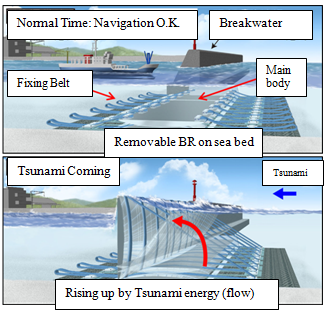AB

Figure 1(A) Image of Hydro-Plane type removable breakwater; (B) Visor Gate in Aji-River.Figure 3 Tsunami profile acting to river gate.

# Experimental condition

Figure 4 shows the tsunami generation basin in Ujikawa Open Laboratory, Kyoto University. In the basin, a solitary wave is generated by a piston type wave generator. A current generator is capable to generate long periodical or uniform and combination flow in the basin as well. The length and width of the channel is 45 and 4m respectively. Another end of channel is a slope of 1/10. In the experiment, the hydro-plane type removable breakwater with a scale of 1/33 is installed on the flat part in the channel. Figure 5(A) shows the cross and plane figure of the installed model inside the tsunami channel. Figure 5(B) shows the overview of the model installment. The model unit is 43cm high, 129cm wide and 4.8cm thick. 3unit is employed in the experiment.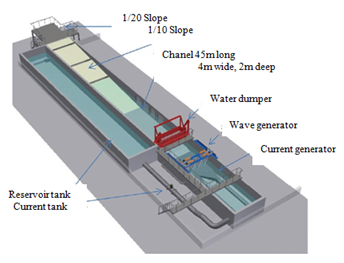Figure 4 Tsunami generator basin in Ujikawa Open Laboratory, Kyoto University.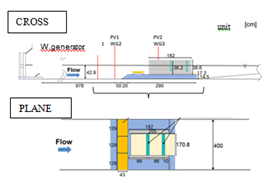Figure 5(A) Settlement of model gate in the basin.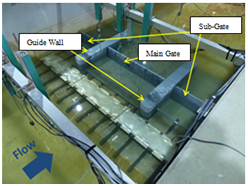Figure 5(B) Overview of the model in the basin.

The input tsunami is divided into three category; Level 1, Level 2 and Level 3. Level 1 is the tsunami which occurs once in several ten years. Level2 occurs once in several hundred from several thousand years. Level 3 corresponds to the mega-earthquake in which predicted all faults collapse in the South-West Japan. The tsunami height at the Aji River gate becomes in model scale 8.8, 10.6 and 15.4cm respectively in Level 1, Level 2 and Level 3 (Figure 3). The peak of run-up tsunami in a river is assumed to become a wave with soliton dispersion. The solitary wave is acted as a representative even in the experimental wave. As the standing wave component with long period is also included in tsunami profile, the long period variable flow is added in the specified cases. Table 1 shows the acting tsunami condition in the experiment. Figure 6 shows the current profiles for tsunami flow components. Table 1 shows the composition of the input tsunami wave and current and their index in the paper as well.

 No. of case Input wave height (cm) Wave Name of case 1 8.8 Soliton *_L10 2 8.8 Soliton +Current(U) *_L101 3 10.6 Soliton *_L20 4 10.6 Soliton +Current(U) *_L201 5 15.4 Soliton *_L30 6 15.4 Soliton +Current(U) *_L301

Table 1 Generated tsunami conditionFigure 6 Current profile for long period component of tsunami.

The removable breakwater is usually employed as it stands vertically on the sea bed. The final position, however, is capable to be adjusted by the length of rubber connection belts. In the experiment, the final position is varied in “Low”, “Middle” and “High”. Figure 7 shows the three final positions of the breakwater. The middle position is the half rising from the sea bottom and it is not perfect standing position. The duration, however, to reach is short and it is not so long to become an active energy dissipater. The distance between the breakwater and gate is changed in two patterns as shown in Figure 8. The left and right side figure correspond to the force and eight times larger than the width of single unit breakwater respectively. Figure 9 shows the position of wave pressure sensors on the gate model. In the analysis, the averaged value of all sensor’s pressure is used as the representative pressure acting on the visor gate.Figure 7 Removable limitation image of each case.Figure 8 Distance between breakwater and gate.Figure 9 Position of wave pressure sensors on visor gate model.

# Experimental result

The reproduction of wave height is calculated employing as (The maximum wave height in WG3(obtained inside the harbor)/(The maximum wave height obtained outside the harbor)). But the basement for gate is inside the harbor and the fare comparison is not capable be done. Herein, the effective height reduction rate is calculated as (reduction rate in “Middle” or “High” position) / (reduction rate in “Low”) The effective wave pressure reduction rate is calculated as follows; (The averaged pressure in “Middle” or “High”) / (The averaged wave pressure in “Low”). Figure 10 shows the variation of the effective reduction wave height for gate height. Figure 11 shows the variation of pressure reduction rate for the gate height. The wave height and pressure becomes reduced in case that the breakwater is adjusted in the position “Middle”. The position “Middle” has a smaller inclination, so the rising motion is faster completed. Figure 12 shows the variation of height reduction for the distance between the gate and breakwater. Figure 13 shows the variation of reduction of wave pressure for the distance. In case that the distance between the breakwater and gate, the influence by the reflection wave become remarkable. The influence of the reflection wave may decrease the reduction effect of the removable breakwater for wave height and wave pressure in comparison with the wider distance. When the distance becomes 175cm, the averaged pressure reduction rate becomes small to 0.74 in a specified case.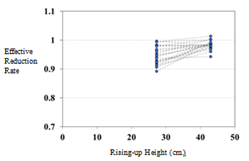Figure 10 Reduction rate for rising-up height.Figure 11 Pressure reduction for rising-up height.Figure 12 Reduction rate for gate distance.

# Proposal of experimental formula

Two kinds of experimental formulae are proposed in this chapter. One is the variation of effective wave height. Another is the averaged wave pressure rate. The both variables are related to the width of breakwater, water depth, wave period etc. Table 2 shows the non-dimensional parameter correlating to the wave height reduction rate and wave pressure reduction rate. Equation (1) shows the correlation of the model experimental wave height and wave height rate. Figure 14 shows the variation of positive non-dimensional wave height and X1. Figure 15 shows the comparison of averaged pressure reduction rate in the experiment and analyzed one from Eq.(3). The estimated curve agrees well to experimentally obtained pressure reduction rate.

$\text{y}=-18.04{X}_{1}^{2}+31.55{X}_{1}-12.80$

${X}_{1}＝{\left(\frac{D}{\lambda }\right)}^{0.03}{\left(\frac{H}{{h}_{0}}\right)}^{0.06}{\left(\frac{T}{2\pi \sqrt{l/3g}}\right)}^{0.05}{\left(\frac{{h}_{B}}{{h}_{0}}\right)}^{-0.08}$  (1)

Here when total water depth becomes larger than 1.68, the X1 is modified as X1’.

$X{\text{'}}_{1}={X}_{1}{\left(\frac{{H}_{3}+{h}_{0}}{{h}_{B}}\right)}^{-0.04}$  (2)

$y=-20.52{X}_{2}^{2}+33.77{X}_{2}-12.89$

${X}_{2}＝{\left(\frac{D}{\lambda }\right)}^{0.09}{\left(\frac{H}{{h}_{0}}\right)}^{0.05}{\left(\frac{T}{2\pi \sqrt{l/3g}}\right)}^{0.14}{\left(\frac{{h}_{B}}{{h}_{0}}\right)}^{-0.14}$  (3)

In case that the breakwater stands –up completely before the wave body reaches to the breakwater, X2 is replaced by X2’.

$X{\text{'}}_{2}={X}_{2}{\left(\frac{{H}_{3}+{h}_{0}}{{h}_{B}}\right)}^{-0.13}$  (4)

 ①Non Dimensional Wave Period $\text{T}/2\text{π}\sqrt{l/3g}$ ②Non Dimensional ${h}_{B}/{h}_{0}$ ③Non Dimensional Wave Height $\text{H}/{h}_{0}$ ④Non Dimensional Distance $\text{D}/\text{λ}$ ⑤Non Dimensional Total Depth $\left({H}_{3}+{h}_{0}\right)/{h}_{B}$ Maximum Rising-up Height of Breakwater ${h}_{B}$ Water Depth ${h}_{0}$ Wave Period T Height of Breakwater l Gravity Acceraration g Maximum Incident wave height H Distance between Breakewater and Gate D Wave Length of Incident Wave $\text{λ}$ Maximum Incident Wve Height ( at WG3) Hz

Table 2 Variable relating the tsunami height and pressure reduction rateFigure 12 Reduction rate for gate distance.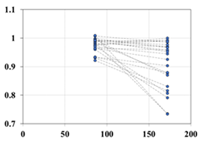Figure 13 Pressure reduction for rising-up height.Figure 14 Estimated and obtained effective wave height reduction rate.Figure 15 Estimated and obtained effective wave pressure reduction rate.

# Conclusion

The many series of fundamental model test to study on applicability of the Fin-type tsunami-energy dissipating gate using a tsunami basin were carried out. The following conclusions were derived.

1. The averaged wave height reduction rate becomes minimum in case of the breakwater height “Middle”
2. The averaged wave pressure reduction rate becomes minimum in case of the breakwater height “Middle”
3. The above two terms become smaller in case that the distance from the gate becomes larger.
4. Experimental formulae are derived to reproduce the variation of wave heights and pressures.

# Acknowledgements

The authors are thankful to the affiliate University for the use of the Laboratory facilities during this study.

# Conflict of interest

The author declares there is no conflict of interest.